## Physics from reason alone

Foundations of physics and/or philosophy of physics, and in particular, posts on unresolved or controversial issues

### Re: Physics from reason alone

So, continuing with where spin comes from in elementary particles. My thought was that there must be some means of virtual particle pairs to avoiding each other along the path from where they pop into existence to where they annihilate each other. It would seem necessary that they curve around each other in some way along the path. And this curvature in their path gives rise to an angular momentum, or spin.

However, I don't actually see a curved path in the math. There is a square of the difference in the space coordinate in the complex exponent of the Gaussian used in the propagator of a particle. But only the difference in the space coordinate is used in the propagator. Can anyone see a curvature implied in the squares of the space and/or time coordinates in the propagator? Or is the spin just some extra property that we assume to exist so that the particle/antiparticle can avoid each other along the way? Thanks.
friend

Posts: 81
Joined: Sat Mar 01, 2014 10:15 am

### Re: Physics from reason alone

friend wrote: Or is the spin just some extra property that we assume to exist so that the particle/antiparticle can avoid each other along the way? Thanks.

Does the spin of an electron in the Standard Model describe something actually turning such that it predicts the phase angle of some reference point that rotates and then returns to a starting point after some period of rotation? Or is it just a vector of some magnitude along the direction of spin but no description of what is spinning with a phase of some angle as it (whatever it is) turns?
friend

Posts: 81
Joined: Sat Mar 01, 2014 10:15 am

### Re: Physics from reason alone

friend wrote:Does the spin of an electron in the Standard Model describe something actually turning such that it predicts the phase angle of some reference point that rotates and then returns to a starting point after some period of rotation? Or is it just a vector of some magnitude along the direction of spin but no description of what is spinning with a phase of some angle as it (whatever it is) turns?

If these particles are indeed point particles, then it seems likely that they are completely symmetrical about their axis of rotation. So there is no feature to serve as a reference with respect to an angle as they spin. In other words, the spin is completely inherent, it does not refer to some feature that is doing the spinning. Every angle would look the same.

So here again we have spin necessary for electrons and positrons so they can propagate. I have to wonder how close am I to getting the speed of light.
friend

Posts: 81
Joined: Sat Mar 01, 2014 10:15 am

### Re: Physics from reason alone

friend wrote:If these particles are indeed point particles, then it seems likely that they are completely symmetrical about their axis of rotation. So there is no feature to serve as a reference with respect to an angle as they spin. In other words, the spin is completely inherent, it does not refer to some feature that is doing the spinning. Every angle would look the same.

So in order for the virtual electron and positron to annihilate when they meet together again, whatever angle each had when they were created, each will have to have rotated at least 180 degrees when they meet together again so that things cancel out. Could this be what is responsible for the spin 1/2 for these particles?
friend

Posts: 81
Joined: Sat Mar 01, 2014 10:15 am

### Re: Physics from reason alone

friend wrote:
Austin Fearnley wrote:Its a while since I read about your ideas. I remember that an implication seemed interestingly like wavefunction collapse of many points in a set to a single point or implication. (Though QM does not use sets.)

As a reminder: I start with individual points and describe each with individual coordinates. (I don't have the dimensions of space or a metric yet.) And like anything else, you describe them using propositions which are either true or not. But now having propositions, we can consider conjunctions and disjunctions of them and how one might imply the other. A space consists of a logical conjunction of all the points in the space. And a conjunction between any two points implies an implication one way in conjunction with the reverse implication. Implication is represented with a complex Gaussian for reasons explained on the website. An implication looks exactly like the propogator (wavefunction) of a particle; the reverse implication is the complex conjugate that then looks like the antiparticle. Both seem to be traveling from the same start and end points at the same time, and since they start from nothing they end in nothing; they cancel each other out. In other words, we have virtual particles popping in and out of existence between every two points in the space that you can imagine, a sea of virtual particles making up the quantum fields through which particles travel.

Austin Fearnley wrote:Does a wavefunction exist in spacetime?

The complex Gaussians that represent implications are functions of time and space. Without a spacetime to begin with, there are no Gaussians.

Austin Fearnley wrote:If it does, then do you have to have every point in the set for an electron wavefunction avoiding every point in the set for a positron? Or is it only the (final) implication that is actually in spacetime and needs its own space (as in Pauli's Exclusion principle).

You may not need the number of dimensions to specify angular momentum. But you do need a metric to specify the magnitude of the radius times the magnitude of the velocity. Is it possible to specify a metric without specifying the dimensionality?

My website is at: logictophysics.com

And the Gaussians are just pulled out of a hat. So your approach is not based on logic, it is based on making arbitrary choices. It does not give formulas which could be used to make predictions which could in principle be wrong so it is not science, it is fantasy. [With all respect, in my personal opinion, etc. I am impressed by your grand research programme, it is a noble enterprise, but for me, it does not come off the ground]
gill1109
Mathematical Statistician

Posts: 2812
Joined: Tue Feb 04, 2014 10:39 pm
Location: Leiden

### Re: Physics from reason alone

gill1109 wrote:And the Gaussians are just pulled out of a hat. So your approach is not based on logic, it is based on making arbitrary choices. It does not give formulas which could be used to make predictions which could in principle be wrong so it is not science, it is fantasy. [With all respect, in my personal opinion, etc. I am impressed by your grand research programme, it is a noble enterprise, but for me, it does not come off the ground]

You're are asking whether the Gaussian is a necessary and unique choice to represent the Dirac delta function. There are three reason why I believe so. First, it has the iterative property that mimics a similar property in the logic. In the logic we have,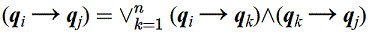.

and this is mimicked by the Dirac delta function,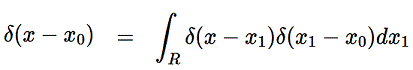.

And the Gaussian representation fulfills this property as shown in the Chapman-Kolmogorov equation,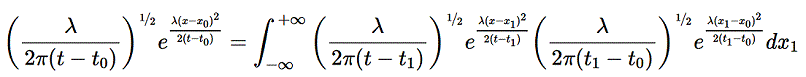.

I've not seen a Chapman-Kolmogorov style equation for any other function used to give a Dirac delta function. I've asked around without response. You might think that ANY function used to give a Dirac delta must also have this iterative property. I'm sure it is my fault for not trying other functions. I guess I was discouraged from doing so because the last equation given above seems like it might take some effort to prove. It would be helpful if someone could show me one other function that also has this iterative property. All I need to see is one.

But even if there were, there's a second reason for choosing the Gaussian form. The Gaussian represents the least complicated. Any other choice would have strange characteristics, sharp or asymmetrical features, that would require explaining. The Gaussian represent a distribution of totally random samples. It is shaped that way because the randomness leaves nothing to explain.

But there's a third reason. The formulation is developed by using very many implications. But in logic, there can be no preference for one implication over another; they all have the same importance and relevance and weight. So whatever function represents an implication, there cannot be any size or value differences depending on which implication is used. When the exponential of the Gaussian become complex, it gives the same absolute value of 1 for every implication. The only difference is a phase.

Then you ask how is this formulation falsifiable? What predictions does it give to test it? Well, firstly it seems to give the Feynman path integral for the wave function. Secondly, it seems to give the number of various particles as specified by the Standard Model. And thirdly it seems to indicate the possibility of deriving the value of the coupling constant, or possibly the masses of the various particles. I'm beginning to wonder if it might be the theory of everything. Only time will tell. However, if you do see an error, please stop me now.

more at: logictophysics.com
friend

Posts: 81
Joined: Sat Mar 01, 2014 10:15 am

### Re: Physics from reason alone

friend wrote:But there's a third reason. The formulation is developed by using very many implications. But in logic, there can be no preference for one implication over another; they all have the same importance and relevance and weight. So whatever function represents an implication, there cannot be any size or value differences depending on which implication is used. When the exponential of the Gaussian become complex, it gives the same absolute value of 1 for every implication. The only difference is a phase.

more at: logictophysics.com

I've shown how the path integral can be derived starting with propositional logic. But I've not shown why this particular formulation is unique or necessary. For that I'd have to show the uniqueness of every step. I was able to introduce numbers, 0 and 1, into the logic by using the Dirac measure as a substitute for implication. In retrospect this seems like an obvious step. But to be honest, I do not know if there is any other way of introducing numbers into the logic. If numbers are necessarily the cardinality of sets, then the most basic numbers would have to be 0 for the empty set, and 1 for a set with only one element. If cardinality is the only way to get numbers out of sets, then it is necessary to use the Dirac measure to express set inclusion, and it's necessary to use set inclusion to express material implication. This would make it necessary to express the conjunction of all points of space with a disjunction of a conjunction of implications that can then be expressed as a sum of a multiple of Kronecker delta functions. This at least allowed me to derive the rule of addition and multiplication and a type of path integral from that. So the question is: are numbers necessarily always the cardinality of sets?

I then migrated to the continuous case by letting the Kronecker delta change to a Dirac delta function. When I express the Dirac delta function as a complex exponential Gaussian, the Feynman Path Integral emerged, at least for a free particle with no potential energy term. I've given some reasons why the complex exponential Gaussian should be chosen for the Dirac delta function. But this is not the same as a necessity. The Feynman Path Integral has been controversial; some question whether the measure of that integral can be mathematically justified. So if I were to show the absolute necessity of using the complex exponential Gaussian in this case, would that get us closer to a mathematical justification of the Path Integral itself? The question I have is whether the complex exponential Gaussian is the only function whose infinite multiple gives a well defined measure that can be integrated. If it is, then this necessitates its use to represent the Dirac delta function.
friend

Posts: 81
Joined: Sat Mar 01, 2014 10:15 am

### Re: Physics from reason alone

friend wrote:
friend wrote:But there's a third reason. The formulation is developed by using very many implications. But in logic, there can be no preference for one implication over another; they all have the same importance and relevance and weight. So whatever function represents an implication, there cannot be any size or value differences depending on which implication is used. When the exponential of the Gaussian become complex, it gives the same absolute value of 1 for every implication. The only difference is a phase.

more at: logictophysics.com

I've shown how the path integral can be derived starting with propositional logic. But I've not shown why this particular formulation is unique or necessary. For that I'd have to show the uniqueness of every step. I was able to introduce numbers, 0 and 1, into the logic by using the Dirac measure as a substitute for implication. In retrospect this seems like an obvious step. But to be honest, I do not know if there is any other way of introducing numbers into the logic. If numbers are necessarily the cardinality of sets, then the most basic numbers would have to be 0 for the empty set, and 1 for a set with only one element. If cardinality is the only way to get numbers out of sets, then it is necessary to use the Dirac measure to express set inclusion, and it's necessary to use set inclusion to express material implication. This would make it necessary to express the conjunction of all points of space with a disjunction of a conjunction of implications that can then be expressed as a sum of a multiple of Kronecker delta functions. This at least allowed me to derive the rule of addition and multiplication and a type of path integral from that. So the question is: are numbers necessarily always the cardinality of sets?

I then migrated to the continuous case by letting the Kronecker delta change to a Dirac delta function. When I express the Dirac delta function as a complex exponential Gaussian, the Feynman Path Integral emerged, at least for a free particle with no potential energy term. I've given some reasons why the complex exponential Gaussian should be chosen for the Dirac delta function. But this is not the same as a necessity. The Feynman Path Integral has been controversial; some question whether the measure of that integral can be mathematically justified. So if I were to show the absolute necessity of using the complex exponential Gaussian in this case, would that get us closer to a mathematical justification of the Path Integral itself? The question I have is whether the complex exponential Gaussian is the only function whose infinite multiple gives a well defined measure that can be integrated. If it is, then this necessitates its use to represent the Dirac delta function.

I don’t understand Feynman path integrals. It looks to me you have replaced one complex (in two senses!) computational approach with another. If it makes you feel good, that’s great! If you can explain it to others, that’s greater still. If it makes in-principle falsifiable predictions then that’s quite exciting. Do carry on. I can’t follow you. I’m just an ordinary statistician. And a mathematician. And not young any more.
gill1109
Mathematical Statistician

Posts: 2812
Joined: Tue Feb 04, 2014 10:39 pm
Location: Leiden

### Re: Physics from reason alone

friend wrote:The Feynman Path Integral has been controversial; some question whether the measure of that integral can be mathematically justified. So if I were to show the absolute necessity of using the complex exponential Gaussian in this case, would that get us closer to a mathematical justification of the Path Integral itself? The question I have is whether the complex exponential Gaussian is the only function whose infinite multiple gives a well defined measure that can be integrated. If it is, then this necessitates its use to represent the Dirac delta function.

For those interested, let me note the issue and a possible solution, or at least a question. The integration measure used in the path integral is: . When n becomes infinite, the measure becomes undefined (at least in some circumstances). There's more information here, and maybe here.

The reason there may be some trouble defining this measure might be because they are looking at it as a single unit of a measure. However, the integration measure is well defined in the Chapman-Kolmogorov equations shown here..
It occurs to me that if we use this equation in an iterative process, then the measure remains well defined for any (at least finite) value of n no matter how large. Is it fair to then say that it is well defined as n approaches (but never reaches) infinity? This is what in fact I did to get the path integral. After all, if the multiple integral always equals the same thing no matter how many times you iterate, then doesn't that mean that the measure must exists if the integral exists?

The question then becomes, what functions can we integrate inside the path integral? I think this amounts to asking if we can let vary from each iteration step to the next. I'd have to see more detail on how to prove the Chapman-Kolmogorov I've shown above. Does anybody know how to prove this equation?
friend

Posts: 81
Joined: Sat Mar 01, 2014 10:15 am

### Re: Physics from reason alone

gill1109 wrote:
friend wrote:
friend wrote:But there's a third reason. The formulation is developed by using very many implications. But in logic, there can be no preference for one implication over another; they all have the same importance and relevance and weight. So whatever function represents an implication, there cannot be any size or value differences depending on which implication is used. When the exponential of the Gaussian become complex, it gives the same absolute value of 1 for every implication. The only difference is a phase.

more at: logictophysics.com

I've shown how the path integral can be derived starting with propositional logic. But I've not shown why this particular formulation is unique or necessary. For that I'd have to show the uniqueness of every step. I was able to introduce numbers, 0 and 1, into the logic by using the Dirac measure as a substitute for implication. In retrospect this seems like an obvious step. But to be honest, I do not know if there is any other way of introducing numbers into the logic. If numbers are necessarily the cardinality of sets, then the most basic numbers would have to be 0 for the empty set, and 1 for a set with only one element. If cardinality is the only way to get numbers out of sets, then it is necessary to use the Dirac measure to express set inclusion, and it's necessary to use set inclusion to express material implication. This would make it necessary to express the conjunction of all points of space with a disjunction of a conjunction of implications that can then be expressed as a sum of a multiple of Kronecker delta functions. This at least allowed me to derive the rule of addition and multiplication and a type of path integral from that. So the question is: are numbers necessarily always the cardinality of sets?

I then migrated to the continuous case by letting the Kronecker delta change to a Dirac delta function. When I express the Dirac delta function as a complex exponential Gaussian, the Feynman Path Integral emerged, at least for a free particle with no potential energy term. I've given some reasons why the complex exponential Gaussian should be chosen for the Dirac delta function. But this is not the same as a necessity. The Feynman Path Integral has been controversial; some question whether the measure of that integral can be mathematically justified. So if I were to show the absolute necessity of using the complex exponential Gaussian in this case, would that get us closer to a mathematical justification of the Path Integral itself? The question I have is whether the complex exponential Gaussian is the only function whose infinite multiple gives a well defined measure that can be integrated. If it is, then this necessitates its use to represent the Dirac delta function.

I don’t understand Feynman path integrals. It looks to me you have replaced one complex (in two senses!) computational approach with another. If it makes you feel good, that’s great! If you can explain it to others, that’s greater still. If it makes in-principle falsifiable predictions then that’s quite exciting. Do carry on. I can’t follow you. I’m just an ordinary statistician. And a mathematician. And not young any more.

The use of path integrals in quantum mechanics was discovered by Feynman. He generalized the principle of least action in classical mechanics, where the action is given as the integral of the Lagrangian. If this integral is minimized, this gives the lagrangian equation of motion, which can be solved for the classical motion of the system. Feynman, a very smart fellow, generalized this principle so that each path has an action, and took the integral over all paths for a quantum mechanical system. He found that this expression can solve quantum mechanical problems, just like Schrodinger's equation but has a different interpretation. What this has to do with Boolian logic escapes me.
jreed

Posts: 176
Joined: Mon Feb 17, 2014 5:10 pm

### Re: Physics from reason alone

jreed wrote:The use of path integrals in quantum mechanics was discovered by Feynman. He generalized the principle of least action in classical mechanics, where the action is given as the integral of the Lagrangian. If this integral is minimized, this gives the lagrangian equation of motion, which can be solved for the classical motion of the system. Feynman, a very smart fellow, generalized this principle so that each path has an action, and took the integral over all paths for a quantum mechanical system. He found that this expression can solve quantum mechanical problems, just like Schrodinger's equation but has a different interpretation. What this has to do with Boolean logic escapes me.

Thanks for the summary. What does physics have to do with logic? Physics has to do with what exists or not. Logic has to do with what is true or false. Both existence or nonexistence and true or false are both binary states. So logic can be used to symbolically represent what exists or not. This is actually quite obvious. For we use propositions, which are either true or false, to describe physical situations which may exist or not. This is simply the correspondence principle used as the definition of truth, that reality is described by propositions which are true. This is the starting premise of my work. It's really quite simple, even advanced high school students can follow along.

I start by recognizing that each point in space is different from every other point in space. We recognize this when we assign different coordinates to each point. But this is actually a logical relationship. For what is being said is that this point here is part of space AND this other point here is part of space AND this still other point is part of space AND... This is expressed as a conjunction (^) of all points as shown next.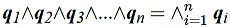,

where each q represents its own point in space. Obviously there is an infinite number of them.

It is then easy to show that a conjunction (^) implies a material implication (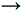) as shown next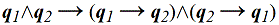.

My website (logictophysics.com) points to a place on the web where you can enter logical expressions and have a truth-table given for it. This makes it easy to check my work.

And if we apply this to the entire conjunction, we get,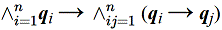.

But I also show on my website that,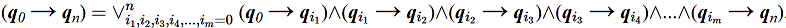.

I slow walk my readers to this equation with simple expressions they can plug into a truth-table generator. All it requires is a little patience. But here you can start to see the beginnings of the path integral. If the conjunctions (^) can be replaced with summations and the disjuctions (V) replaced by multiplications and the implications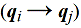replaced by transition amplitudes, then the path integral will result. The question is how to do this.

One reason why you may not understand what Boolean logic has to do with physics is because you don't understand how numbers can be introduced to logic. Logic and numbers in your mind are completely divorced from each other. So physics, which is mathematical, cannot possibly be connected with symbolic logic. I remedy this by using the Dirac measure to get the Kronecker delta. The Dirac measure assigns a value of 1 if the element is included in the set A, and is 0 otherwise.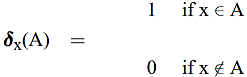Set inclusion represents implication very well. For if the entire set is said to exist, then so can its element be said to exist. But just because an element may exist does not mean that the entire set exists. So we can replace implication in the logic with the Dirac measure. And this brings in numbers into the logic.

But in the logic, a proposition used as a premise in one implication can just as easily be used as a conclusion in some other implication. So the set, A, of the Dirac measure can be shrunk down to the size of an element so that the set A can be represented by a number as well. Then we get the Kronecker delta,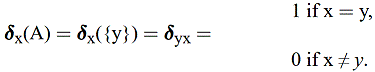Now that we have numbers, how do we get the math of addition and multiplication? Consider the following equation,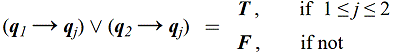.

If j is between 1 and 2, then the expression is true. If j is outside this range, then it is false. This is because for either j=1 or j=2, the expression reduces to (q1q1)V(q2q1) = T V F = T, or it reduces to (q1q2)V(q2q2) = F V T = T. But if j is outside this range, then both (q1qj) and (q2qj) are false. So if the analogy with the Kronecker delta is to be upheld, then we are looking for a math expression for conjunction (let's call it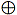for now) such that the following expression results. Remember, we can replace T for 1 since T = (qxqx) which is replace by = 1.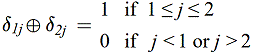When we replace the implications with Kronecker deltas and the T with 1 and the F with 0, the following table results for the various conditions for j.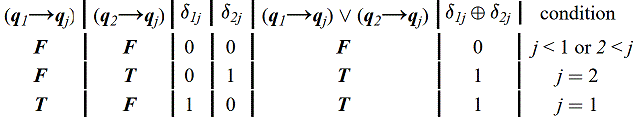Notice that there is never the case where j = 1 and j = 2 at the same time. So we will never have the case where = 1 and = 1 at the same time. It is obvious from this table thatshould be represented by the math operator of addition +.

How do we find the math operator for conjunction? Consider the logic expression below: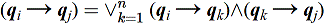.

If we replace the implications with Kronecker deltas and the disjunctions with addition, then we get,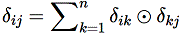,

with,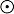, as some yet unknown math operator for conjunction. With n=1 in the last expression, k can only have the value of 1, and the last expression reduces to,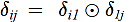. With i or j any value other than 1, this expression is 0. And we get the following table for the specified conditions: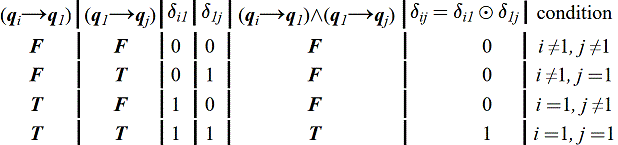.

Obviously,represents the math operator of multiplication. And putting it all together, the logic expression,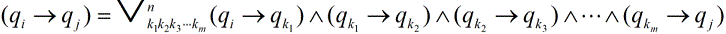,

becomes the math expression,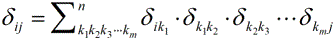.

I think it is a typical step to go from the discrete case with the Kronecker delta to the continuous case with the Dirac delta function. From there it's a matter of finding reasons why to select the complex exponential Gaussian to represent the Dirac delta function. But when this is done, the Feynman Path Integral results. I've been debating gill1109 as to why the complex exponential Gaussian function should be chosen for the Dirac delta function. He thinks I've pulled it out of the air because it works. I think I have more obvious reason for selecting it.

I include a little more detail in my website at: logictophysics.com
friend

Posts: 81
Joined: Sat Mar 01, 2014 10:15 am

### Re: Physics from reason alone

friend wrote:OK, I have a few misstatements that I have to correct. I wrote " If the conjunctions (^) can be replaced with summations and the disjuctions (V) replaced by multiplications". This should be, " If the conjunctions (^) can be replaced with multiplications and the disjuctions (V) replaced by summations". Sorry about that. It seems I read what I meant to write and not what I actually wrote. I only catch these mistakes if I let enough time pass before rereading it so I can forget what I intended to write, which is difficult. Here is a corrected version.

What does physics have to do with logic? Physics has to do with what exists or not. Logic has to do with what is true or false. Existence and nonexistence are mutually exclusive binary states just as true and false are mutually exclusive binary states. So logic can be used to symbolically represent what exists or not. This is actually quite obvious. For we use propositions, which are either true or false, to describe physical situations which may exist or not. This is simply the correspondence principle used as the definition of truth, that reality is described by propositions which are true. This is the starting premise of my work. It's really quite simple, even advanced high school students can follow along.

I start by recognizing that each point in space is different from every other point in space. We recognize this when we assign different coordinates to each point. But this is actually a logical relationship. For what is being said is that this point here is part of space AND this other point here is part of space AND this still other point is part of space AND... This is expressed as a conjunction (^) of all points as shown next.,

where each q represents its own point in space. Obviously there is an infinite number of them.

It is then easy to show that a conjunction (^) implies a material implication () as shown next.

My website (logictophysics.com) points to a place on the web where you can enter logical expressions and have a truth-table generated for it. This makes it easy to check my work.

And if we apply this to the entire conjunction, we get,.

But I also show on my website that,.

I slow walk my readers to this equation with simple expressions they can plug into a truth-table generator. All it requires is a little patience. But here you can start to see the beginnings of the path integral. If the conjunctions (^) can be replaced with multiplications and the disjuctions (V) replaced by summations and the implicationsreplaced by transition amplitudes, then the path integral will result. The question is how to do this.

One reason why you may not understand what Boolean logic has to do with physics is because you don't understand how numbers can be introduced to logic. Logic and numbers in your mind are completely divorced from each other. So physics, which is mathematical, cannot possibly be connected with symbolic logic. I remedy this by using the Dirac measure to get the Kronecker delta. The Dirac measure assigns a value of 1 if the element is included in the set A, and is 0 otherwise.Set inclusion represents implication very well. For if the entire set is said to exist, then so can its element be said to exist. But just because an element may exist does not mean that the entire set exists. So we can replace implication in the logic with the Dirac measure. And this brings in numbers into the logic.

But in the last logic expression above, a proposition used as a premise in one implication can just as easily be used as a conclusion in some other implication. So let the set, A, of the Dirac measure be shrunk down to near the size of an element so that the set A can be represented by a number as well. Then we get the Kronecker delta,Now that we have numbers, how do we get the math of addition and multiplication? As I explain on my website, a proposition, q, can be written as Pq, which is true if the object q has the property P and is false if q does not have the property P. But the property, P, has the extension P={q1,q2,q3,...}, the set of all such objects that have that property. This means that q is true if (q ∈ P) and q is false if (q ∉ P). When I use set inclusion to represent implication, (Pq) is equal to (q ∈ P) for which the Dirac measure is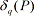. In terms of the extension of P={q1,q2,q3,...qn}, then (Pqj) = T only if 1jn, and is F if j1 or jn. If P has only one element, qk, then the implication, qjqk=T only for j=k and is F otherwise. Then we have the following:.

If j is either 1 or 2, then the expression is true. If j is outside this range, then it is false. This is because for either j=1 or j=2, the expression reduces to (q1q1)V(q2q1) = T V F = T, or it reduces to (q1q2)V(q2q2) = F V T = T. But if j is outside this range, then both (q1qj) and (q2qj) are false. So if the analogy with the Kronecker delta is to be upheld, then we are looking for a math expression for disjunction (let's call itfor now) such that the following expression results. Remember, we can replace T for 1 since T = (qxqx) which is replace by = 1.When we replace the implications with Kronecker deltas and the T with 1 and the F with 0, the following table results for the various conditions for j.Notice that there is never the case where j = 1 and j = 2 at the same time. So we will never have the case where = 1 and = 1 at the same time. It is obvious from this table thatshould be represented by the math operator of addition +.

How do we find the math operator for conjunction? Consider the logic expression below:.

If we replace the implications with Kronecker deltas and the disjunctions with addition, then we get,,

with,, as some yet unknown math operator for conjunction. With n=1 in the last expression, k can only have the value of 1, and the last expression reduces to,. With i or j any value other than 1, this expression is 0. And we get the following table for the specified conditions:.

Obviously,represents the math operator of multiplication. And putting it all together, the logic expression,,

becomes the math expression,.

I think it is a typical step to go from the discrete case with the Kronecker delta to the continuous case with the Dirac delta function. From there it's a matter of finding reasons why to select the complex exponential Gaussian to represent the Dirac delta function. But when this is done, the Feynman Path Integral results. There is some debate as to why the complex exponential Gaussian function should be chosen for the Dirac delta function. I have shown on my website some obvious reasons for selecting it.

I include a more detail on my website at: logictophysics.com
friend

Posts: 81
Joined: Sat Mar 01, 2014 10:15 am

### Re: Physics from reason alone

I think you have found a formal analogy between certain expressions in formal logic, and certain expressions in the ‘theory’ of Feynman path integrals. But I don’t find this surprising. I wonder if it is *useful*? Could you use the analogy to make new, testable, conjectures about path integrals?
gill1109
Mathematical Statistician

Posts: 2812
Joined: Tue Feb 04, 2014 10:39 pm
Location: Leiden

### Re: Physics from reason alone

gill1109 wrote:I think you have found a formal analogy between certain expressions in formal logic, and certain expressions in the ‘theory’ of Feynman path integrals. But I don’t find this surprising. I wonder if it is *useful*? Could you use the analogy to make new, testable, conjectures about path integrals?

You're right. I am drawing an analogy between logic and physics by using the Dirac measure. I don't know that I have a complete mathematical analysis of propositional logic. We'll have to ask someone more skilled in analysis than I am. My framework seems to be more than an analogy; it seems to be a direct mapping from one domain (logic) to the other (math). Isn't it curious how I come up with addition for disjunction and multiplication for conjunction. It works for the numbers 0 and 1. Have I included enough information to use mathematical induction for any numbers? I've not seen anywhere else where they derive addition of probabilities for alternative possibilities or multiplication of probabilities for a conjunction of possibilities - the sum and product rule of probabilities.

I used the iterations of logical expressions to find what seems to be the various particles of the Standard Model. See logictophysics.com/StandardModel.html. This is all derived from assuming that points in space are in conjunction with each other. So one thing my model predicts is if there is space, then we should always have these particles, it would seem even during inflation and even during any phase transition from the false vacuum to the true vacuum. Some would suggest that particles did not exit yet during inflation, and others suggest that a collapse of the false vacuum would possibly give us a whole new set of particles. And others would suggest that there may be other universes that have a different set of particles and laws. But my work seems to indication that if there is space with points in conjunction, then we have the set of particles of the Standard Model.
friend

Posts: 81
Joined: Sat Mar 01, 2014 10:15 am

### Re: Physics from reason alone

friend wrote:
gill1109 wrote:I think you have found a formal analogy between certain expressions in formal logic, and certain expressions in the ‘theory’ of Feynman path integrals. But I don’t find this surprising. I wonder if it is *useful*? Could you use the analogy to make new, testable, conjectures about path integrals?

You're right. I am drawing an analogy between logic and physics by using the Dirac measure. I don't know that I have a complete mathematical analysis of propositional logic. We'll have to ask someone more skilled in analysis than I am. My framework seems to be more than an analogy; it seems to be a direct mapping from one domain (logic) to the other (math). Isn't it curious how I come up with addition for disjunction and multiplication for conjunction. It works for the numbers 0 and 1. Have I included enough information to use mathematical induction for any numbers? I've not seen anywhere else where they derive addition of probabilities for alternative possibilities or multiplication of probabilities for a conjunction of possibilities - the sum and product rule of probabilities.

I used the iterations of logical expressions to find what seems to be the various particles of the Standard Model. See logictophysics.com/StandardModel.html. This is all derived from assuming that points in space are in conjunction with each other. So one thing my model predicts is if there is space, then we should always have these particles, it would seem even during inflation and even during any phase transition from the false vacuum to the true vacuum. Some would suggest that particles did not exit yet during inflation, and others suggest that a collapse of the false vacuum would possibly give us a whole new set of particles. And others would suggest that there may be other universes that have a different set of particles and laws. But my work seems to indication that if there is space with points in conjunction, then we have the set of particles of the Standard Model.

Boole worked out probability *and* logic. Of course there may me a one-to-one correspondence between some parts of one formalism and some parts of another. If you can *prove* that it is indeed one-to-one then you can transpose theorems in the one field to theorems in the other. This is already big business in mathematics, using theorems about theorems to prove new theorems. Category theory does this.

You say that logic is one domain, math is another. I would say that formal logic is a field within maths. Formal calculations of path integrals are another field within maths. Mathematicians love connecting fields to one another, basically that is seeing a pattern of patterns. We humans love to see patterns. It’s what our minds are for, what our brains do. Another thing is seeing connections with the real world. e.g. path integrals are connected to data seen in particle scattering experiments. Formal logic is connected to verbal reasoning about the world. A lot of mathematicians have worked with some success in connecting path integrals calculations to previously formalised parts of quantum physics. Von Neumann invented a huge part of the theory of Hilbert spaces in order to bring other people’s informal or heuristic calculations into main stream maths. Matrix mechanics and wave mechanics turned out to be mathematically isomorphic. At least, some main features of both. Dirac went further still, making QM relativistic and in the way discovering “spin” which later was observed in experiments.

Some patterns we find do have predictive powers. Many don’t, and they get forgotten.
gill1109
Mathematical Statistician

Posts: 2812
Joined: Tue Feb 04, 2014 10:39 pm
Location: Leiden

### Re: Physics from reason alone

Two points I found interesting.

Friend wrote:
So one thing my model predicts is if there is space, then we should always have these particles, it would seem even during inflation and even during any phase transition from the false vacuum to the true vacuum. Some would suggest that particles did not exit yet during inflation, and others suggest that a collapse of the false vacuum would possibly give us a whole new set of particles.

My thoughts draw on three ideas. 1. Penrose's CCC model (cyclic conformal cosmology) 2. My work on Rasch as used in psychometrics and 3 My preon model.

Much depends on what you mean by a particle and, on what you mean by space, and on relativity (or where you are when making a measurement).

Penrose's model has the beginning of a cycle of the universe at a single point where the only contents are photons (or, at least, bosons). Bosons like to be together in the same state (a BEC or Bose Einstein Condensate). Very sociable and hence they like being at a single point together. But it is often said that photons are not matter. At any rate, photons are very different to matter particles (electrons and quarks etc) and the maths used for bosons and fermions are different. So consider all the photons together at the start of the universe at a point. Well, I am not sure that it is a point in the universe's spacetime. Using a universe-as-particle model, then that point is probably in an outer universe, just as an electron when measured is located at a point in our spacetime.

After some disturbance the bosons start to convert to fermions. Imagine zillions of bosons coming crashing down into the new universe as fermions. Like coins in a single pile crashing on to a table. But fermions obey Pauli's exclusion principle. They must have their own states and their own spaces. Hence the universe expands in size. But Pensose and the Rasch model say that there cannot be a metric of space if there are no fermions. The bosons are not sufficient alone to provide a metric of space. That is why the cycle of the universe ends when all the fermions have converted back to bosons. So the cycles are basically bosons --> fermions --> bosons --> fermions etc etc.

The next point is, can we have space existing without a metric? This is very similar to the issue of 'where is an electron when it is not at a measurement?' that is when the electron is in field form rather than particle form. I can apply the CCC model to an electron measurement. At a measurement the contents (yes, preon content, a Standard Model sacrilege) of an electron are at a single point in our spacetime and that corresponds via CCC to the electron contents being in purely bosonic form. Ironically, in this model, Standard Model particles are matter particles in between measurement when we do not know where they are but have bosonic contents when they are measured and we do know where they are in our spacetime. That's a kind of role reversal of matter and non-matter behaviours and comes about due to the differents in relativity: whether we are describing the particle from within or from outside it.

My preon model implies that each and every particle in the Standard Model contains ALL the properties of multidimensional spaces. Some properties cancel out in some particles as my model has the additive properties built up of +1 and -1. So an electron has colour charged preons within it but they all cancel out with anticolour properties leaving it colour neutral.

So at the CCC beginning of a universal cycle, there is no space metric but all the information about the spatial dimensions are contained in the photons. BUt they have no metric. As soon as fermions form, space expands and the metric is founded. It is believed by some that the metric is formed by bosons measuring the fermions in say entangled pairs. But, whatever the truth of that, Penrose and Rasch imply that you need the fermions to make a metric. And my preon model argues that space is embedded in preons as associations of spaces somehow glued together with some new force acting over very small distances within the elementary particles.

And that means that one always gets the same old spaces in every cycle. And the same old forces ec. I do not know enough to know why the CCC mode must be conformal. I suspect that only matters if you are tryng to prove a link between one cycle and the next by searching in the early heavens of the universe. It seems much more likely to me that 'conformal' is unnecessary, unless I am missing something.
Friend wrote:
I've been debating gill1109 as to why the complex exponential Gaussian function should be chosen for the Dirac delta function. He thinks I've pulled it out of the air because it works. I think I have more obvious reason for selecting it.

I am not sure why this is important? If you take a sledgehammer slamming horizontally at near the speed of light to flatten any statistical pdf then you get a very thin vertical line. Does it really matter what the pdf distribution was originally? I suppose relativity matters as if you are viewing from inside the distribution then the distribution might never change shape from your viewpoint. But, looked at from outside , the distribution will always be a boringly identical vertical line. With my preon model, I could think what the red colour charge dimension would look like from inside it. But from us outside it it is either a +1 or a -1. A very boring(?) loss of information but I suppose a lot is gained for us with the colour dimensions. Do we really use only the +/-1 data or are the underlying colour dimensions having an effect on us too. If so how? Are the underlying distributions important or is it just the thin vertical quantised smears that play a role on us?
Austin Fearnley

### Re: Physics from reason alone

gill1109 wrote:Boole worked out probability *and* logic. Of course there may me a one-to-one correspondence between some parts of one formalism and some parts of another. If you can *prove* that it is indeed one-to-one then you can transpose theorems in the one field to theorems in the other. This is already big business in mathematics, using theorems about theorems to prove new theorems. Category theory does this.

It seems obvious that set inclusion can represent implication. And it seems that this applies to any size set, so it seems obvious we can shrink the set down to the near size of a point. This allows me to use the Dirac measure to represent implication. And when the set is the size of a point, this gives me the Kronecker delta, which allowed me to actually define addition and multiplication in terms of this mapping.

However, this brings up a problem. We seem to loose some of the properties of implication. Normally, the implication, (qx -> qy), is true only when qx is false or when qy is true. But in the Kronecker representation, (qx -> qy) is true only when qx=qy because this give . Also, there is the problem that an implication equals its reverse, (qx -> qy) = (qy -> qx), which is not the case in logic, but is the case with the Kronecker since .

Perhaps the first can be fixed by shrinking the Kronecker delta to its infinitesimal version, the Dirac delta. Then we don't know if the point is in the set of the Dirac measure or not. Both the size of the set and the distance between the point and the set are infinitesimal, and all possibilities become available again of whether the point is actually in the set or not.

The second can be fixed by making the math representation of implication be the complex conjugate of its reverse implication. I don't know how to do this with the Kronecker delta. But I do know how to do this with the Dirac delta, just use the complex Gaussian form of the Dirac delta function.

Whitehead and Russel seem to have derived arithmetic from logic. This took quite a few pages of math to accomplish. I seem to have made a shortcut using the Dirac measure. I don't know what this says about my method or theirs. They came up with the numbers two and higher. I just assume that since it works for 0 and 1, it works for larger numbers as well, a type of mathematical induction. So I'm not really sure how reliable of a map I have from logic to math. At this point, I'm noting the similarities I've gained to the formulations of physics, and I'm asking for help.
friend

Posts: 81
Joined: Sat Mar 01, 2014 10:15 am

### Re: Physics from reason alone

Austin Fearnley wrote:So consider all the photons together at the start of the universe at a point....The bosons are not sufficient alone to provide a metric of space.

Matter can stand still in space. But photons are defined as moving... at the speed of light. If there is no expanse of space for photons to move within, then there can be no photons. Right?

There is also the possibility that the acceleration of the universe increases as the density of the universe thins out. Perhaps the universe thins out so much that space expands so fast that there is another round of inflation. In other words, the universe did not come from a point, but came from acceleration due to a lack of particles.
friend

Posts: 81
Joined: Sat Mar 01, 2014 10:15 am

### Re: Physics from reason alone

Friend wrote:
Matter can stand still in space. But photons are defined as moving... at the speed of light. If there is no expanse of space for photons to move within, then there can be no photons. Right?

Well, IMO as an amateur, from our viewpoint the photon is created by a fermion, then travels alone at speed c, and then is annihilated by a fermion. If the fermions are all gone then there is no means of creation of new, nor destruction of old, photons. There may be other ways of creation/destruction that I am overlooking, but I do not think so. Penrose talks of only photons surviving to the CCC nodes but I am unclear about other bosons such as gluons.

From a photon's viewpoint, it travels at infinite speed. That is not quite true as it does not travel through time so we cannot divide by zero time to get a proper velocity, but let's say it is infinite. This is similar to dirac delta territory. If the time elapsed from the photon's point of view is infinitesimal rather than zero, then the photon merely has a 'near infinite' speed from its own point of view.

So we cannot have new photons created nor old ones destroyed at the CCC node. Again we are in delta dirac territory. What about the situation just before the end of the universe cycle? In my Rasch work analogy, I would have the universe's metric gradually being destroyed rather than destroyed in one single dirac-delta-like instant.

So what happens to photons at or near the CCC node which cannot be destroyed but still exist? And there are a lot of them as all the fermions have been converted into bosons/photons in the late stage of the universe's cycle prior to the CCC node event. And if unitarity exists then all the photons from the previous CCC node re-exist at the next CCC node. (Not necessarily completely true with my preon model as there is more that one way of making a photon when different preons can be aggregated to make a photon - so not necessarily exactly the 'same' photons. But all the preons will still exist.)

This is a little like the warnings not to mistake a higgs field for a higgs boson. There is a difference between the photon as a field and the photon as a particle. Penrose says that at the end of cycle, ie at the CCC node, all the photons share the same state in a condensate. With almost zero energy. Wavelength is inversely related to energy and so the photons have almost (I will skip the dirac Delta analogy) infinite wavelength. Basically, their fields are analogous to a completely flat ocean surface of unlimited scope. There is not enough energy around to make a surf wave (ie photon 'particle').

{Q. What happens to the higgs fields at the end of cycle of the CCC universe?}
Austin Fearnley

### Re: Physics from reason alone

Austin Fearnley wrote:Well, IMO as an amateur, from our viewpoint the photon is created by a fermion, then travels alone at speed c, and then is annihilated by a fermion. If the fermions are all gone then there is no means of creation of new, nor destruction of old, photons. There may be other ways of creation/destruction that I am overlooking, but I do not think so. Penrose talks of only photons surviving to the CCC nodes but I am unclear about other bosons such as gluons.

Interesting stuff, but off topic for this thread. Let me suggest that you start your own thread. I will will save my comment for then.
friend

Posts: 81
Joined: Sat Mar 01, 2014 10:15 am

PreviousNext